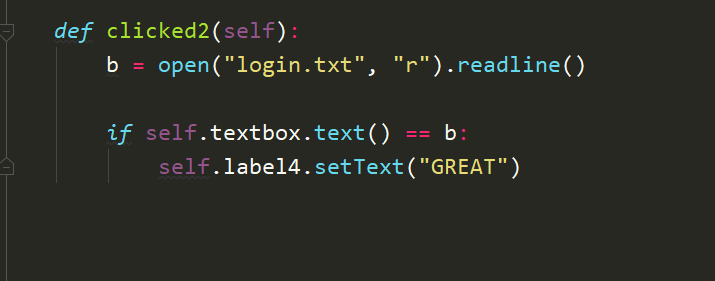# Variable from notepad problem

• Hello dear friends, i have made here simple function and if statement.

Variable b is equal to "a" and i put in my text box "a". But label do not change. When i replace in if statement variable b with string "a" then it is working. So my question is what should i add there for get it work ? I tried str(b) but not working is there anything else iam missing ?

b is equal to "a"
and i put in my textbox "a"
but label do not change• Hi,

To have an idea of their content, you should print both values. You may have a subtle difference between the two.

• I n the login.txt file, the value of b is "some text\n". As I see, here is a QlineEdit. You can try:

``````if self.textbox.text() == b.rstrip("\r\n"):
``````